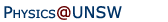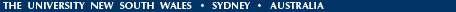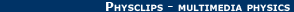Relativity in brief... or in detail..

Where does E = mc2 come from? Energy in Newtonian mechanics and in relativity

Under relativity, the laws of physics may be the same for two observers with relative motion, but they sometimes look unfamiliar to those of us who are used to putting kinetic energy = ½ mv2. In this animation, a rocket engine does work at a constant rate, i.e. it produces constant power. (This is not the usual behaviour or rockets, but it means that we can see what increasing the kinetic energy does to speed and momentum under classical and relativistic mechanics.) We discuss this animation further below.

Another way of writing Newton's second law in classical mechanics is the work energy theorem. By integrating the resultant force F that acts on a body with respect to the distance over which the centre of mass moves,

one obtains the important result that the work done by F equals the change in the quantity ½mv2, a quantity that we call the kinetic energy. That's the theorem. As you might expect, things are a bit more complicated in relativity. Let's start with Newton's second law, that states the force is equal to the time rate of change in momentum:We integrate to get the work done: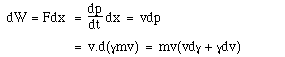Now we need some expressions to do the substitutions:We need to integrate with respect to both v and γ, so it's helpful to write: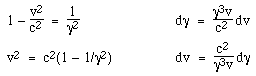and substitution in our integrand gives: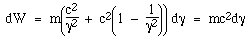From here we get the expression for the kinetic energy, K, in Special Relativity:This is a very important expression, so we should check that, at low speeds, it corresponds to the classical expression. Using the expansion valid for small v/c: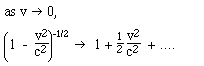soas shown in the graph at right - the purple line is mc2+½mv2. Ah, that's reassuring. But it also shows us Einstein's speed limit. In the classical expression, v is proportional to the square root of K. In principle, v could be as large as one liked. In relativistic mechanics, however, (γ - 1) increases linearly with the kinetic energy. And γ goes to infinity as v goes to c. Hence, a finite amount of energy can never accelerate a body to the speed of light. So now let's look at equation (*) again:Where did the 1 on the right hand side come from? It's the starting value of the integral. Now all of the terms in this equation are energies. When γ > 1, we have non-zero kinetic energy. So, if we think of γmc2 as the total energy of body, and write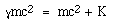then (γ - 1)*mc2 is the kinetic energy, and 1*mc2 is an energy that a body has when v = 0 and γ = 1. Remember the proper time and proper length of module 4. These were the time and length of a body measured in its own frame. So we could writewhere E0 = mc2 is the proper energy of a body — the energy that it has, even when it is not moving. So that famous equation, E0 = mc2, has come from identification of the constant of integration in the relativistic version of the work-energy theorem. Given that it's such an important equation, it's interesting to ask: Is this identification of the proper energy a derivation or an inspired guess on Einstein's part? My judgment would be somewhere between the two. We are getting used to large numbers, but let's remember that c2 = 9.0 x 1016 m2s-2 = 9.0 x 1016 J/kg. Yes, c2 has units of joules per kilogram, as we can see from the familiar expression Kclassical = ½mv2. So the conversion of one kilogram mass would liberate nearly 1017 joules. Ouch! (By the way, this is not a feature restricted to nuclear energy: See the discussion of binding energy, and the example below.)
A moderately large power station might provide power at 1 GW or 109 joules/second. To provide this power continuously for a year requires 3 x 1016 joules. So, in such a power station, about 300 grams of matter would disappear each year. (Because this is a small amount, the actual accounting would be complicated by a range of processes that occur to the rods.)

In a coal fired power station with the same continuous power output, 300 grams of matter would also disappear. Yes, if you could gather all the CO2, H2O, soot, nitrides and other products of the power station (ah, if only we could gather them!) then we'd have 300 grams less than the coal and air that we reacted in the furnaces.

As another example, consider a bomb that reduces the total mass of its fuel. Suppose that the blast from this bomb pushes the atmosphere out of the way in a hemisphere of radius R. (It is said that, in the Manhattan project, one of the physicists used the displacement of pieces of debris to estimate the yield after the first test. A simple calculation that one can do in one's head gives the yield:

The work done against the atmosphere is atmospheric pressure times 2πR3/3, the volume of the hemisphere. Suppose that R is 100 m, substitution shows that this requires the loss of 2 micrograms of matter. Again, it doesn't matter whether it is a chemical or nuclear bomb. Fortunately, extremely large chemical bombs are very hard to deliver. I regret to say that nuclear bombs small enough to be carried in a backpack or fired from a cannon, but with huge destructive power, have been developed by several countries.

Now let's examine our two travellers.

Notice Einstein's speed limit: the Space Ship Newton can accelerate indefinitely, but the SS Einstein approaches c as the energy put into it goes to infinity. On the other hand, the momentum rises more rapidly with input energy under relativity.

For more about Newtonian and Galilean mechanics, see Physclips: Mechanics with animations and film clips.Home | Summary | Quiz | Credits School of Physics - The University of New South Wales Sydney, Australia 2052 © School of Physics UNSW 2052 Disclaimer feedback